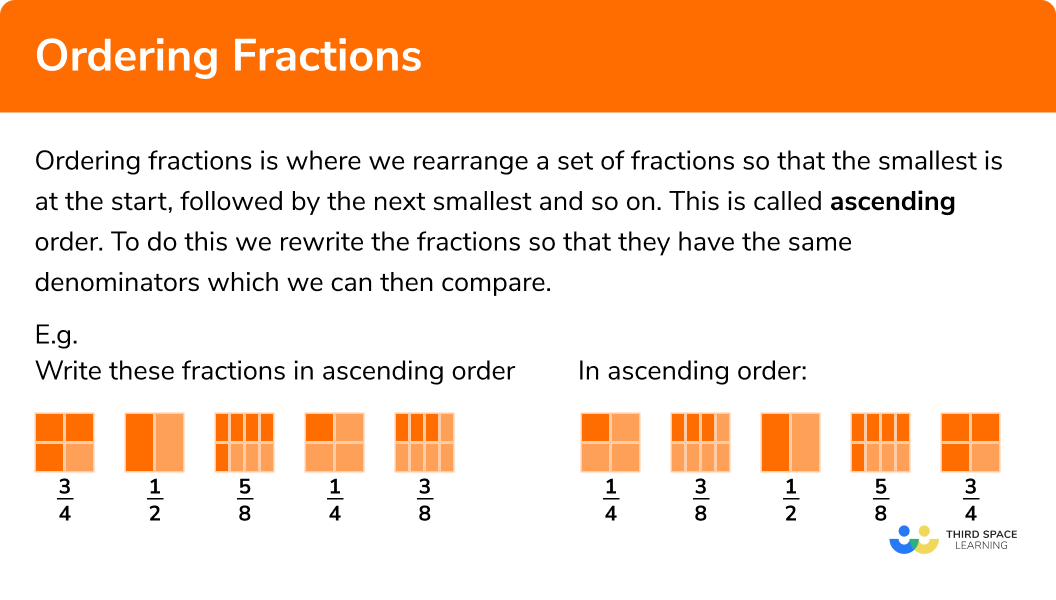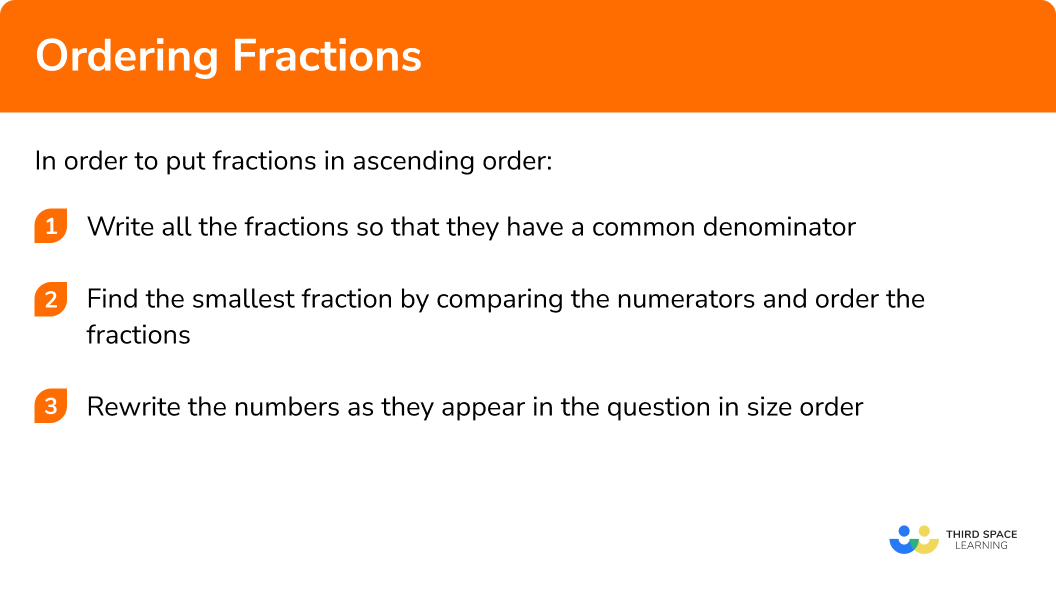# Ordering‌ ‌Fractions‌

Here we will learn about ordering fractions.
There are also ordering fractions worksheets based on Edexcel, AQA and OCR exam questions, along with further guidance on where to go next if you’re still stuck.

## What is ordering fractions?

Ordering fractions is where we rearrange a set of fractions so that the smallest is at the start, followed by the next smallest and so on. This is called ascending order.

To do this we rewrite the fractions so that they have the same denominators which we can then compare.

We can order any type of fraction including proper fractions, improper fractions and mixed numbers.

E.g.

Write these fractions in ascending order:

In ascending order:

### What is ordering fractions?## How to order fractions

In order to put fractions in ascending order:

1. Write all the fractions so that they have a common denominator
2. Find the smallest fraction by comparing the numerators and order the fractions
3. Rewrite the numbers as they appear in the question in size order

### Explain how to put fractions in ascending order in 3 steps### Related lessons on fractions

Ordering fractions is part of our series of lessons to support revision on fractions. You may find it helpful to start with the main fractions lesson for a summary of what to expect, or use the step by step guides below for further detail on individual topics. Other lessons in this series include:

## Ordering fractions examples

### Example 1: ordering proper fractions

Write the following fractions in order of size:

$\frac{3}{4} \quad \quad \frac{1}{2} \quad \quad \frac{5}{6} \quad \quad \frac{7}{12}$

1. Write all the fractions so that they have a common denominator

The fractions have different denominators.
The denominators of the fractions are 2, 4, 6 and 12. 2, 4 and 6 go into 12.
The lowest common multiple is 12, so we can convert the fractions so they have a common denominator of 12.

$\frac{3\times3}{4\times3}=\frac{9}{12}$

$\frac{1\times6}{2\times6}=\frac{6}{12}$

$\frac{5\times2}{6\times2}=\frac{10}{12}$

2Find the smallest fraction by comparing the numerators and order the fractions

Here are the fractions with their common denominator of 12

$\frac{9}{12} \quad \quad \frac{6}{12} \quad \quad \frac{10}{12} \quad \quad \frac{7}{12}$

Writing them in size order would give

$\frac{6}{12} \quad \quad \frac{7}{12} \quad \quad \frac{9}{12} \quad \quad \frac{10}{12}$

3Rewrite the numbers as they appear in the question in size order

$\frac{6}{12} \quad \quad \frac{7}{12} \quad \quad \frac{9}{12} \quad \quad \frac{10}{12}$

$\frac{1}{2} \quad \quad \;\; \frac{7}{12} \quad \quad \;\frac{3}{4} \quad \quad \; \frac{5}{6}$

### Example 2: ordering proper fractions

Write the following fractions in order of size:

$\frac{11}{30} \quad \quad \frac{4}{15} \quad \quad \frac{2}{5} \quad \quad \frac{1}{3}$

The fractions have different denominators.
The denominators of the fractions are 3, 5, 15 and 30.  3, 5 and 15 go into 30
The lowest common multiple is 30, so we can convert the fractions so they have a common denominator of 30.

$\frac{4\times2}{15\times2}=\frac{8}{30}$

$\frac{2\times6}{5\times6}=\frac{12}{30}$

$\frac{1\times10}{3\times10}=\frac{10}{30}$

Here are the fractions with their common denominator of 30

$\frac{11}{30} \quad \quad \frac{8}{30} \quad \quad \frac{12}{30} \quad \quad \frac{10}{30}$

Writing them in size order would give

$\frac{8}{30} \quad \quad \frac{10}{30} \quad \quad \frac{11}{30} \quad \quad \frac{12}{30}$

$\frac{8}{30} \quad \quad \frac{10}{30} \quad \quad \frac{11}{30} \quad \quad \frac{12}{30}$

$\frac{4}{15} \quad \quad \;\frac{1}{3} \quad \quad \;\frac{11}{30} \quad \quad \;\frac{2}{5}$

### Example 3: ordering improper fractions and mixed numbers

Write the following fractions in order of size:

$\frac{7}{4} \quad \quad 1\frac{1}{2} \quad \quad 1\frac{2}{3} \quad \quad \frac{29}{24}$

We can either write all the fractions as improper fractions or as mixed numbers. It is simpler to write them as mixed numbers and concentrate on the fractional part of the mixed number.

$\frac{29}{24}=1\frac{5}{24} \quad \quad \quad \frac{7}{4}=1\frac{3}{4}$

The fractions have different denominators.
The denominators of the fractions are 2, 3, 4 and 24. 2, 3 and 4 go into 24
The lowest common multiple is 24, so we can convert the fractions so they have a common denominator of 24.

$1\frac{3\times6}{4\times6}=1\frac{18}{24}$

$1\frac{1\times12}{2\times12}=1\frac{12}{24}$

$1\frac{2\times8}{3\times8}=1\frac{16}{24}$

Here are the fractions with their common denominator of 24

$1\frac{18}{24} \quad \quad 1\frac{12}{24} \quad \quad 1\frac{16}{24} \quad \quad 1\frac{5}{24}$

Writing them in size order would give

$1\frac{5}{24} \quad \quad 1\frac{12}{24} \quad \quad 1\frac{16}{24} \quad \quad 1\frac{18}{24}$

$1\frac{5}{24} \quad \quad 1\frac{12}{24} \quad \quad 1\frac{16}{24} \quad \quad 1\frac{18}{24}$

$\frac{29}{24} \quad \quad \;\;1\frac{1}{2} \quad \quad \;\;\; 1\frac{2}{3} \quad \quad \;\; \frac{7}{4}$

### Example 4: ordering improper fractions and mixed numbers

Write the following fractions in order of size:

$\frac{12}{5} \quad \quad 2\frac{3}{10} \quad \quad 2\frac{7}{20} \quad \quad \frac{9}{4}$

We can either write all the fractions as improper fractions or as mixed numbers. It is simpler to write them as mixed numbers and concentrate on the fractional part of the mixed number.

$\frac{12}{5}=2\frac{2}{5} \quad \quad \quad \frac{9}{4}=2\frac{1}{4}$

The fractions have different denominators.
The denominators of the fractions are 4, 5, 10 and 20. 4, 5 and 10 go into 20
The lowest common multiple is 20, so we can convert the fractions so they have a common denominator of 20.

$2\frac{2\times4}{5\times4}=2\frac{8}{20}$

$2\frac{3\times2}{10\times2}=2\frac{6}{20}$

$2\frac{1\times5}{4\times5}=2\frac{5}{20}$

Here are the fractions with their common denominator of 20

$2\frac{8}{20}\quad \quad 2\frac{6}{20} \quad \quad 2\frac{7}{20} \quad \quad 2\frac{5}{20}$

Writing them in size order would give

$2\frac{5}{20} \quad \quad 2\frac{6}{20} \quad \quad 2\frac{7}{20} \quad \quad 2\frac{8}{20}$

$2\frac{5}{20} \quad \quad 2\frac{6}{20} \quad \quad 2\frac{7}{20} \quad \quad 2\frac{8}{20}$

$\frac{9}{4} \quad \quad \;\;\;2\frac{3}{10} \quad \quad \;2\frac{7}{20} \quad \quad \;\;\frac{12}{5}$

### Example 5: ordering fractions and decimals

Write the following fractions in order of size:

$\frac{3}{4} \quad \quad 0.55 \quad \quad \frac{1}{2} \quad \quad 0.6$

When there is a mixture of decimals and fractions it is sometimes easier to write them all as decimals.  Some fractions and their decimal equivalents may be well known, otherwise we may need to work out a division.

$\frac{3}{4}=3\div4=0.75$

$\frac{1}{2}=1\div2=0.5$

Here are the numbers as decimals. To help with comparing it is a good idea to put in a zero in the hundredths column for 0.5 and 0.6. We can compare the hundredths.

$0.75 \quad \quad 0.55 \quad \quad 0.50 \quad \quad 0.60$

Writing them in size order would give

$0.50 \quad \quad 0.55 \quad \quad 0.60 \quad \quad 0.75$

$0.50 \quad \quad 0.55 \quad \quad 0.60 \quad \quad 0.75$

$\frac{1}{2} \quad \quad \;\;\;0.55 \quad \quad \; 0.6 \quad \quad \;\; \frac{3}{4}$

### Example 6: ordering fractions and decimals

Write the following numbers in order of size:

$0.67 \quad \quad \frac{2}{3} \quad \quad 0.603 \quad \quad \frac{5}{8}$

When there is a mixture of decimals and fractions it is sometimes easier to write them all as decimal fractions (decimals). Some fractions and their decimal equivalents may be well known, otherwise we may need to work out a division.

$\frac{2}{3}=2\div3=0.666…$

$\frac{5}{8}=5\div8=0.625$

Here are the numbers as decimals. To help with comparing it is a good idea to put in a zero in the thousandths column for 0.67 so we can compare the hundredths.

$0.670 \quad \quad 0.666… \quad \quad 0.603 \quad \quad 0.625$

Writing them in size order would give

$0.603 \quad \quad 0.625 \quad \quad \quad 0.666… \quad \quad 0.670$

$0.603 \quad \quad 0.625 \quad \quad 0.666… \quad \quad 0.670$

$0.603 \quad \quad \;\;\;\frac{5}{8} \quad \quad \;\; \; \; \;\frac{2}{3} \quad \quad \; \; \;\;\; 0.67$

### Common misconceptions

• Changing the numbers into the same type of fractions

It is much easier to change the fractions so that they have a common denominator and then compare the numerators.

• Use decimals if the common denominator is too tricky to find

Sometimes finding a common denominator can be very difficult so it can be easier to convert the fractions to decimals and compare them instead.

• Smallest to largest or largest to smallest?

Usually “in size order” means from smallest to largest.  But the question might want you to put the numbers in descending order from largest to smallest.

E.g.
Write these numbers in order of size from largest to smallest:

$\frac{4}{7} \quad \quad\frac{11}{14}\quad \quad \frac{1}{2}$

Convert the fractions so they have the same denominator:

$\frac{8}{14} \quad \quad \frac{11}{14} \quad \quad \frac{7}{14}$

Write the fractions in order of size from largest to smallest:

$\frac{11}{14} \quad \quad\frac{8}{14} \quad \quad \frac{7}{14}$

$\frac{11}{14} \quad \quad \frac{4}{7} \quad \quad \frac{1}{2}$

### Practice ordering fractions questions

1. Write these numbers in order of size:2. Write these numbers in order of size:3. Write these numbers in order of size:4. Write these numbers in order of size:5. Write these numbers in order of size:6. Write these numbers in order of size:### Ordering fractions GCSE questions

1.   Here are four fractions:

Write the fractions in order of size.

Starting with the smallest fraction.

(2 Marks)

(1)

(1)

2. Here are four fractions:

Write the fractions in order of size.

Starting with the smallest fraction.

(2 Marks)

(1)

(1)

3. Place the following numbers in order of size, smallest first:

(2 Marks)

(1)

(1)

## Learning checklist

You have now learned how to:

• Write fractions in order of size

## Still stuck?

Prepare your KS4 students for maths GCSEs success with Third Space Learning. Weekly online one to one GCSE maths revision lessons delivered by expert maths tutors.

Find out more about our GCSE maths tuition programme.